# Linear Function Equation

Theandcoordinates of points on a straight line have a numerical pattern. There are three numerical patterns to observe on this line:Remember that the first number in a coordinate pair is for, the second is for, that is:.

• The points are plotted such that thecoordinates are going up in ones;
• Thecoordinates are going up ins;

More importantly, there is a pattern between the two numbers in each ordered pair. That is, thecoordinate is four times thecoordinate then add one.

Take the point. Notice,.

Also,. Notice,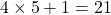.

This calculation works for all the coordinate pairs on the line.

We can capture this calculation with an equation: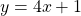Notice that the number we multiplyby is 4. This is the same number thecoordinates are going up in (when thecoordinates go up in ones). We use the letterto represent this number (the multiplier); and we call it the slope because the multiplier determines how steep the line is.

As long as we know two points on a line, we can calculate the slope and then figure out the equation of the line.

To calculate slope,, we can use:or the formulaOnce we know the slope, we know that the equation of the line begins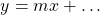If one of the points we know lies on theaxis, that is, the point has, we call it theintercept of the line. This is the number that we complete the equation with.

Example: Determine the equation of the line that goes through the pointsWe only need to use two points to find the equation. Let’s usebecause this is theintercept and.That tells us thatand so our equation begins.

Theintercept has coordinatesand so our equation finishes with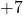We check with some other coordinate pairs:

Use point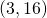:Use point:.

Practice:

Test out on Slope y intercept

# Any Two Points

To find the equation of a line using any two points, we begin by calculating the slope with the formulaWe then substitute theandcoordinates of one known pointand the slopeinto the equationto calculate the value.

Example: Find the equation of the line that goes through the points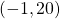,, and.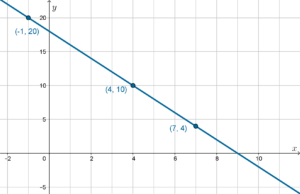Solution: We need only two points to calculate the equation. Let’s useand.

First, calculate slope:Second, calculateusing slope, and any point. Let’s use.We now haveand, therefore the equation of the line is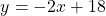which could also be written asjust by changing the order of the terms.

Practice:

# A more efficient formula

If we streamline the process of calculating, we arrive at the formulaThis is sometimes known as the ‘point/slope’ formula.

Using the same example as before, calculate the equation of the line that goes through the pointsandWe need to calculate the slopein the same way:However, to calculate the equation we substituteand choose any known point for the. Let’s useagain:Why it works

This formulais derived as follows:Now substitute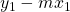in place ofin the formula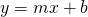and rearrange:Use any method to calculate the equation given the coordinates of one point and the slope: